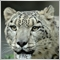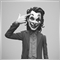# seperate negative values from positive values883

Hello everyone,

I am trying get negative numbers stored separately from positive numbers over a 15 minute timeframe.

Question: How do I do this? Is there an in built function for this?

```void statistics()
{
shift=0;pos=0;
for(shift=0;shift<=26;shift++)
{
CCI[pos]  =iCCI(Symbol(),PERIOD_M15,14,PRICE_CLOSE,shift);
pos++;
}
}```30496

GrumpyDuckMan:

Hello everyone,

I am trying get negative numbers stored separately from positive numbers over a 15 minute timeframe.

Question: How do I do this? Is there an in built function for this?

try this

```int statistics() {
int pos = 0;
for(int shift=26; shift>=0; --shift) {
cci = iCCI(_Symbol,PERIOD_M15,14,PRICE_CLOSE,shift);
if(cci<0) pos++;
}
return pos;
}```